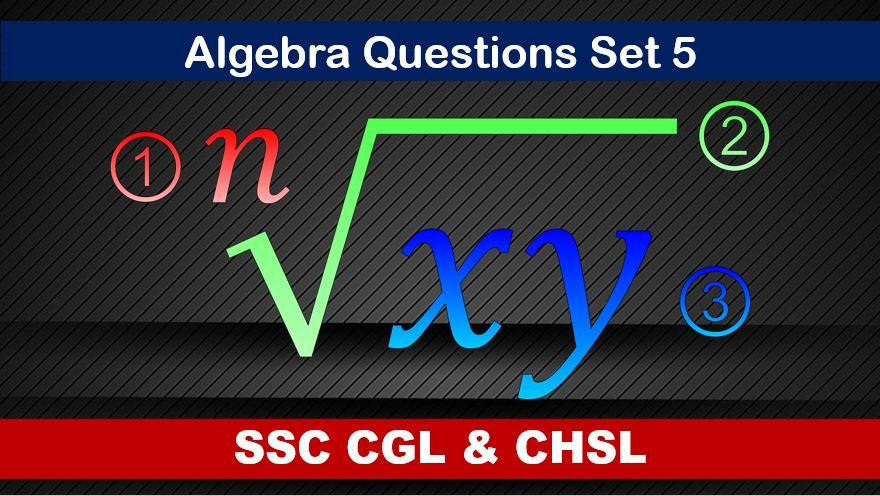Thursday, August 5, 2021
Home > algebra > SSC CGL Algebra Questions and Solutions Set 5

# SSC CGL Algebra Questions and Solutions Set 5SSC CGL CHSL Algebra
Algebra Questions Sets
Set 1Set 2Set 3Set 4Set 5
Set 6

SSC CGL Algebra Questions and Solutions Set 5 : Ques No 1

If 2x – 1/2x = 6, then the value of x2 + 1/16x2 is

Options:

A. 19/2

B. 17/2

C. 16/3

D. 15/2

Solution:

SSC CGL Algebra Questions and Solutions Set 5 : Ques No 2

If a, b, c are real numbers and a + b + c = 0, then the value of (a2 + b2 + c2 ) / (b2 – ca) is

Options:

A. 3

B. 2

C. 1

D. 0

Solution:

SSC CGL Algebra Questions and Solutions Set 5 : Ques No 3

If a3 – b3 – c3 – 3abc = 0, then which of the following is correct?

Options:

A. a = b = c

B. a + b + c = 0

C. a + c = b

D. a = b + c

Solution:

SSC CGL Algebra Questions and Solutions Set 5 : Ques No 4

If the expression 3a(3a + 2c) – 4b(b + c) has a factor of (3a – 2b), then the other factor is

Options:

A. 2(a + b + c)

B. 3a + 2b + 2c

C. 3a – 2b + 2c

D. 3a + 2b – 2c

Solution:

SSC CGL Algebra Questions and Solutions Set 5 : Ques No 5

If the average of x and 1/x (x is non-zero) is M, then the average of x2 and 1/x2 is

Options:

A. 1 – M2

B. 1 – 2M

C. 2M2 – 1

D. 2M2 + 1

Solution:

SSC CGL Algebra Questions and Solutions Set 5 : Ques No 6

If a/(1 – a) + b/(1 – b) + c/(1 – c) = 1, then the value of 1/(1 – a) + 1/(1 – b) + 1/(1 – c) is

Options:

A. 1

B. 2

C. 3

D. 4

Solution:

SSC CGL Algebra Questions and Solutions Set 5 : Ques No 7

Which of the following is a factor of x2 + 9y2 – z2 + 6xy?

Options:

A. x – 3y + z

B. x – 3y – z

C. 3x + y – z

D. x + 3y – z

Solution:

SSC CGL Algebra Questions and Solutions Set 5 : Ques No 8

If x – 1/x = 5, then the value of x2 + 1/x2 is

Options:

A. 5

B. 25

C. 27

D. 23

Solution:

SSC CGL Algebra Questions and Solutions Set 5 : Ques No 9

If x2 + y2 + 1/x2 + 1/y2 = 4, then the value of x2 + y2 is

Options:

A. 2

B. 4

C. 8

D. 16

Solution:

SSC CGL Algebra Questions and Solutions Set 5 : Ques No 10

If x = 3 + 2√2, then the value of (√x – 1/√x) is

Options:

A. 1

B. 2

C. 2√2

D. 3√3

Solution:

SSC CGL Algebra Questions and Solutions Set 5 : Ques No 11

If a, b, c are real numbers such that a2 + b2 + c2 = 2(a – b – c) – 3, then the value of 2a – 3b + 4c is

Options:

A. -1

B. 0

C. 1

D. 2

SSC CGL Algebra Questions and Solutions Set 5 : Ques No 12

If (x – 3)2 + (y – 5)2 + (z – 4)2 = 0, then the value of x2/9 + y2/25 + z2/16 is

Options:

A. 12

B. 9

C. 3

D. 1

SSC CGL Algebra Questions and Solutions Set 5 : Ques No 13

If the expression (x2 + x + 1) is written in the form of (x + 1/2)2 + q2, then the possible values of q are

Options:

A.1/3

B.sqrt(3)/2

C.2/sqrt(3)

D.1/2

SSC CGL Algebra Questions and Solutions Set 5 : Ques No 14

If a2 – 4a – 1 = 0, then the value of a2 + 1/a2 + 3a – 3/a is

Options:

A. 25

B. 30

C. 35

D. 40